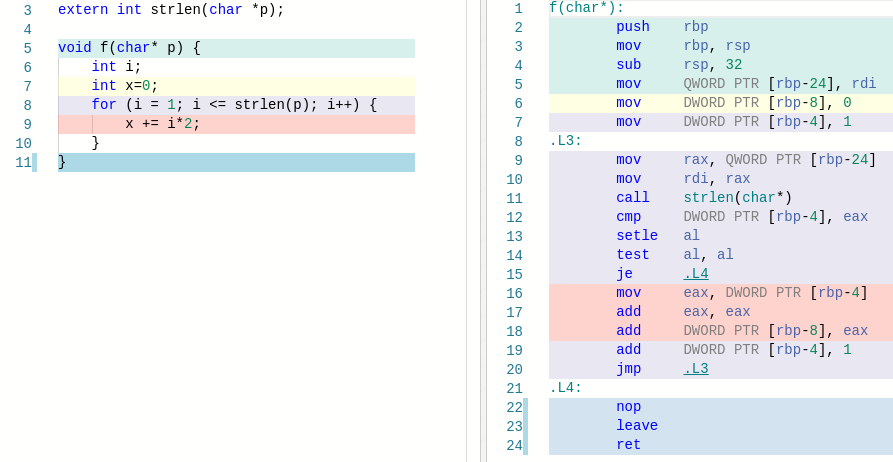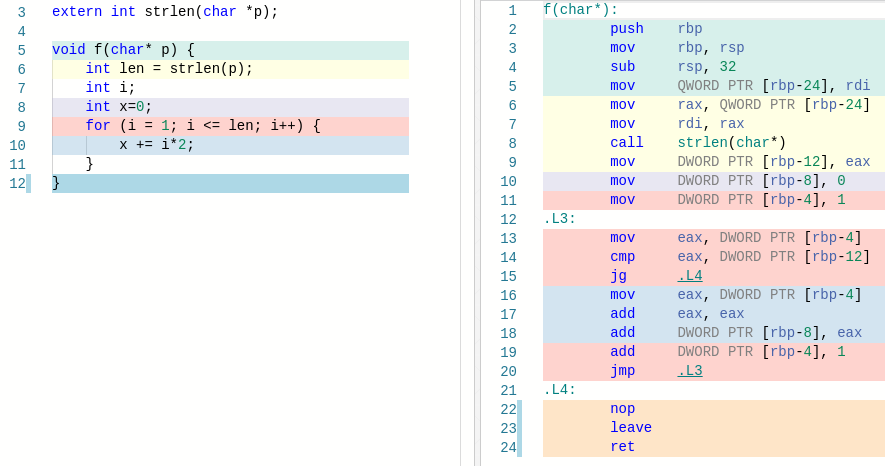# C 语言性能优化：移除循环体中的重复运算extern int strlen(char *p);void f(char* p) {    int i;    int x=0;    for (i = 1; i <= strlen(p); i++) {        x += i*2;    }}extern int strlen(char *p);void f(char* p) {    int len = strlen(p);    int i;    int x=0;    for (i = 1; i <= len; i++) {        x += i*2;    }}## 评论